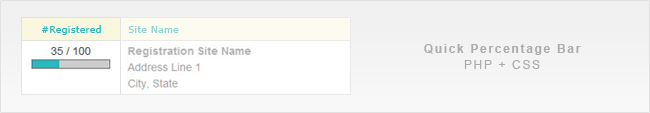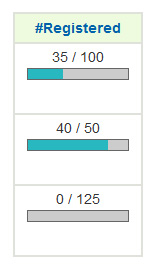If you have some PHP numbers you need to work with, creating a percentage bar is incredibly simple. Calculate the percentage, and then set a width on a DIV that can then be styled.

## Get the Percentage in PHP and Making the Bar

The easiest way to calculate a percentage is to divide the given amount by the total amount, and then multiply by 100. For some reason, I always remember percentages as a cross multiplication. Maybe it is the visual nature of resolving them on paper that clicks. So solving for x, you get:

x / 100 = given / total
x * total = 100 * given
x = (100 * given) / total.

First, the total number (\$max) and the given current number are needed (\$value). In this example, I was using a maximum number of registered users and the number of users currently registered. Then the number as the width of a colored div is echo’d, nested within a grey div. I added in the scale variable in case anyone using the script wants to make it wider than 100 pixels.

## The PHP

```// Testing numbers. Replace with your own.
\$value = 40;
\$max = 150;
\$scale = 1.0;

// Get Percentage out of 100
if ( !empty(\$max) ) { \$percent = (\$value * 100) / \$max; }
else { \$percent = 0; }

// Limit to 100 percent (if more than the max is allowed)
if ( \$percent > 100 ) { \$percent = 100; }```

## The CSS

Styles the grey background and the colored bar inside it.

```.percentbar { background:#CCCCCC; border:1px solid #666666; height:10px; }
.percentbar div { background: #28B8C0; height: 10px; }```

## The Results

Here’s what this looks like on the site I created this for. It was a little extra addition to a time-sensitive pro-bono project, and this was an easy solution.1.Curmudgeon says:

You have an error in this. In the main section, you assign a class of “bargraph” but in the CSS code, you call it “.percentbar”.

2.Josh says:

Thanks. Fixed.

3.Stephen Smith says:

I REALLY don’t understand the percent calculation method used here, but here is my completed function:

function showprogress(\$decimal, \$width) {
echo ”;
echo ‘
‘;
}

It takes \$dec as an already calculated percent in decimal form.

4.VampDiva says:

Really quick, and extremely simple. Thanks!

5.ace300 says:

Nice and simple. Thank you.
Note:
You have an error in your syntax. In the second ‘div’ element, the closing ‘php’ tag is ass-about…

Here:
<div style="width:<?php echo round(\$percent * \$scale); ?`<`px;”>

•Josh says:

I think there may have been a WordPress formatting mistake. I’ve replaced with a GIST.